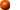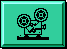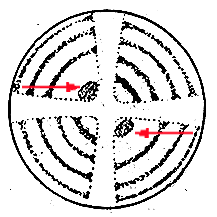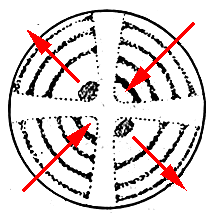Determination of the optic sign of crystals

The interference figure is used to determine the optic group (positive or negative) to which crystals belong.

The interference figure is observed as the compensating plates are introduced. Depending on whether the waves have additive effects (retardation) or subtractive effects (compensation), the directions of vibrations of the slow and fast components of the mineral and of the compensating plate can be determined and thus it is possible to establish the corresponding optic sign.

Use of accesory plates

Son varios los compensadores que se usan, según las características de la figura de interferencia.

1st order red compensator

Mica plate compensator

Quartz wedge compensator1st order red compensator

This plate introduces a retardation equivalent to 1st order red. The subtractive effects (compensation) are represented by 1st order yellow (or orange), whilst additive effects (retardation) are represented by 2nd order blue (or green).When there exist isochromatic curves, instead of analysing the colour changes in a particular quadrant, the colours of two adjacent quadrants are compared (e.g. 1 with 2) and it is apparent that in one, the predominant colours are greens and blues, whilst in the other they are yellows and oranges.For minerals with moderate birefringence, the quadrants show a narrow grey area next to the centre of the cross (which corresponds to the first isochromatic greyish curve) in which it is easy to see whether the colour rises to blue or falls to yellow when the red compensator is introduced.This is highly recommended for interference figures without isochromatic curves.Mica plate compensator

Mica compensating plate with a retardation of 1/4l.

This is highly recommended for interference figures with isochromatic curves.

In the quadrants where there is a coincidence of fast-fast and slow-slow (retardation), the effect of this compensator is that the isochromatic curves move towards the centre, (because they receive additive effects from the compensator, the waves do not have to travel so far inside the crystal to produce the same retardation), whilst when subtractive effects (compensation) occur (fast-slow, slow-fast) the isochromatic curves move towards the periphery of the field.In the quadrants where subtractive effects occur, dark points appear in the centre of the field which are easily recognisable.Quartz wedge compensator

As the wedge is gradually introduced, the isochromatic curves move towards the centre in those quadrants in which additive effects occur, whilst they move outwards in those where subtractive effects occur.This is highly recommended for interference figures with numerous isochromatic curves (very anisotropic minerals).

Index | Introduction | PPL | XPL orthos | XPL conos | Previous | Next | Top